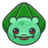首页   注册   登录
V2EX = way to explore
V2EX 是一个关于分享和探索的地方

V2EX  ›  推广

# 一次 Serverless 架构改造实践：基因样本比对

wxxshu · 2017-12-07 18:23:41 +08:00 · 1066 次点击

Serverless 是一种新兴的无服务器架构，使用它，开发者只需专注于代码，无需关心运维、资源交付或者部署。

1. 一个成熟的基因对比算法（ Python 实现，运行一次的时间花费为 2 秒）
2. 2020 个基因样本文件（每个文件的大小为 2M，可以直接作为算法的输入）
3. 一台 8 核心云主机

#### 基因检测服务

``````.
├── relation.py
└── samples
├── one.sample
└── two.sample
``````

`relations.py` 代码如下：

``````import sys

def relationship_algorithm(human_sample_one, human_sample_two):
# it&#39;s a secret
return result

if __name__ == &quot;__main__&quot;:
length = len(sys.argv)
# sys.argv is a list, the first element always be the script&#39;s name
if length != 3:
sys.stderr.write(&quot;Need two samples&quot;)
else:
# read the first sample
with open(sys.argv, &quot;r&quot;) as sample_one:
# read the second sample
with open(sys.argv, &quot;r&quot;) as sample_two:
# run the algorithm
print relationship_algirithm(sample_one_list, sample_two_list)
``````

``````➜ python relation.py ./samples/one.sample ./samples/two.sample
➜ 0.054
``````

#### 我们接到了如下业务需求

``````2000 * 20 = 40000 （次）
``````

``````40000 （次） * 2 （秒）= 80000 （秒）
80000 （秒）/ 60.0 / 60.0 ≈ 22.2 （小时）
``````

``````22.2 / 8 ≈ 2.76 （小时）
``````

### 介绍一种 Serverless 产品：UGC

UCloud General Compute### 针对此 Serverless 架构的改造

1. 改造算法中元数据从「文件输入」改为「标准输入」，输出改为「标准输出」
2. 开发客户端构造 HTTP 请求，并提高并发

#### 1. 改造算法输入输出

① 改造输入为 `stdin`

``````cat ./samples/one.sample ./samples/two.sample | python relation.py
``````

``````import sys

# 这里的 mystdin 包含 ./samples/one.sample ./samples/two.sample 的全部内容，无分隔，实际使用可以自己设定分隔符来拆分
``````

② 将算法的输出数据写入 `stdout`

``````# 把标准输入拆分为两个 sample
sample_one, sample_two = separate(mystdin)

# 改造算法的输出为 STDOUT
def relationship_algorithm(sample_one, sample_two)
# 改造前
return result
# 改造后
sys.stdout.write(result)
``````

#### 2. 客户端与并发

``````imageName = &quot;cn-bj2.ugchub.service.ucloud.cn/testbucket/relationship:0.1&quot;
token = tokenManager.getToken() # SDK 有现成的

# summitTask 构造 HTTP 请求并将镜像的 STDOUT 打成 tar 包返回
response = submitTask(imageName, token, data)
``````

``````import tarfile
import io

def untar(data):
tar = tarfile.open(fileobj=io.BytesIO(data))
for member in tar.getmembers():
f = tar.extractfile(member)
with open(&#39;result.txt&#39;,&#39;a&#39;) as resultf:
resultf.write(strs)
``````

``````sample_2000_list, sample_20_list = get_sample_list()
``````

``````import itertools

all = list(itertools.product(sample_2000_list, sample_20_list))

assert len(all) == 40000
``````

``````def worker(two_file_tuple):
sample_one_dir, sample_two_dir = two_file_tuple

with open(sample_one_dir) as onef:
with open(samle_two_dir) as twof:

data = one_data + two_data
response = summitTask(imageName, token, data)
untar(response)
``````

``````import gevent.pool
import gevent.monkey
gevent.monkey.patch_all() # 猴子补丁

pool = gevent.pool.Pool(200)
pool.map(worker, all)
````````````40000 * 2 = 80000 （秒）
80000 / 200 = 400 （秒）
``````• 免运维
• 高可用
• 按需付费
• 发布简单
1. 免运维 -- 因为你没有服务器了...

2. 高可用 -- Serverless 服务一般依托云计算的强大基础设施，任何模块都不会只有单点，都尽可能做到跨可用区，或者跨交换机容灾，而且本次使用的服务有一个有趣的机制：同一个任务，你提交一次，会被多个节点执行，如果一个计算节点挂了，其他节点还可以正常返回，哪个先执行完，先返回哪个。

3. 按需付费 -- 文中说每个算法执行一次花费单核心 CPU 时间 2 秒，我们直接算一下花费

``````2000 * 20 * 2 = 80000 （秒）
80000 / 60 / 60 / 24.0 = .93 （小时）
.93 （小时） * .09 （元） * 1 （核心） ~= .08 （元）
``````

1. 发布简单 -- 因为使用 Docker 作为载体，所以它是语言无关的，而且发布也很快，代码写好直接上传镜像就好了，至于灰度，客户端 imageName 指定不同版本即可区分不同代码了

1 回复  |  直到 2017-12-08 09:43:52 +08:001 l0o0   2017-12-08 09:43:52 +08:00 知乎上帖子没缩近，这里总算有缩近了。